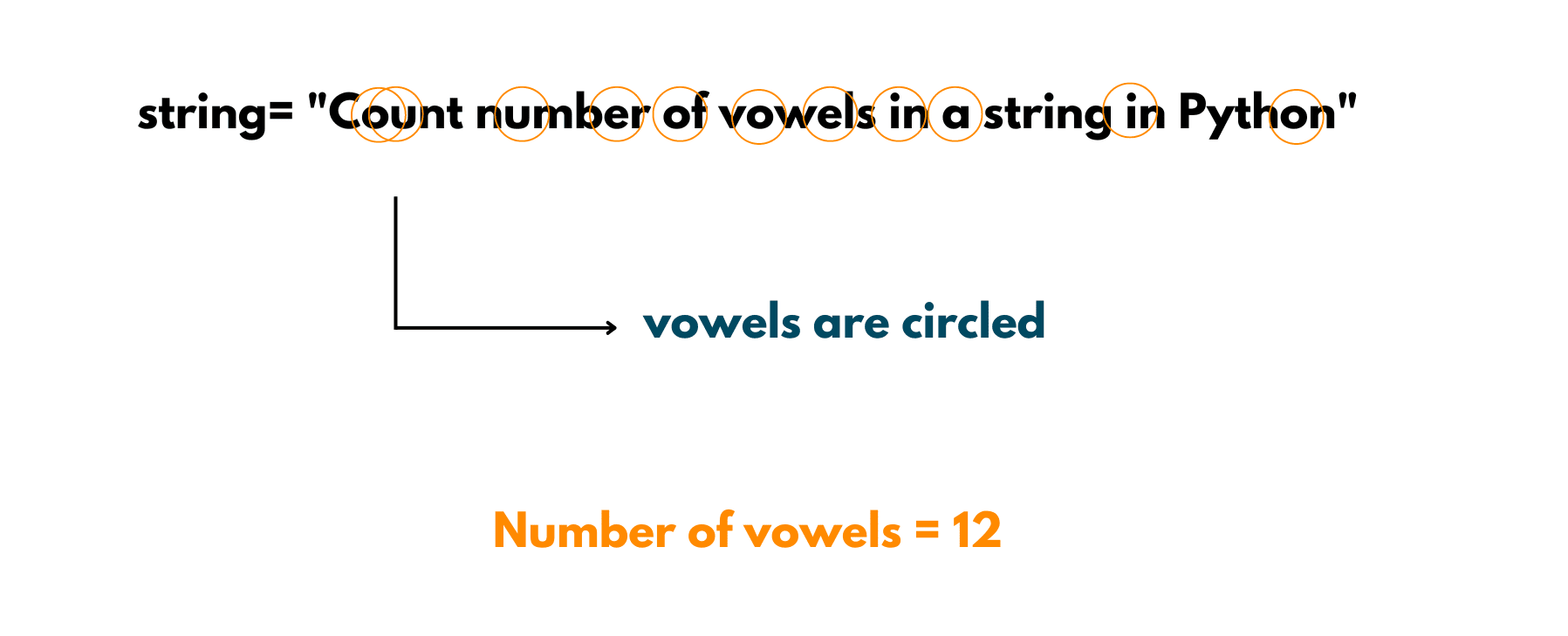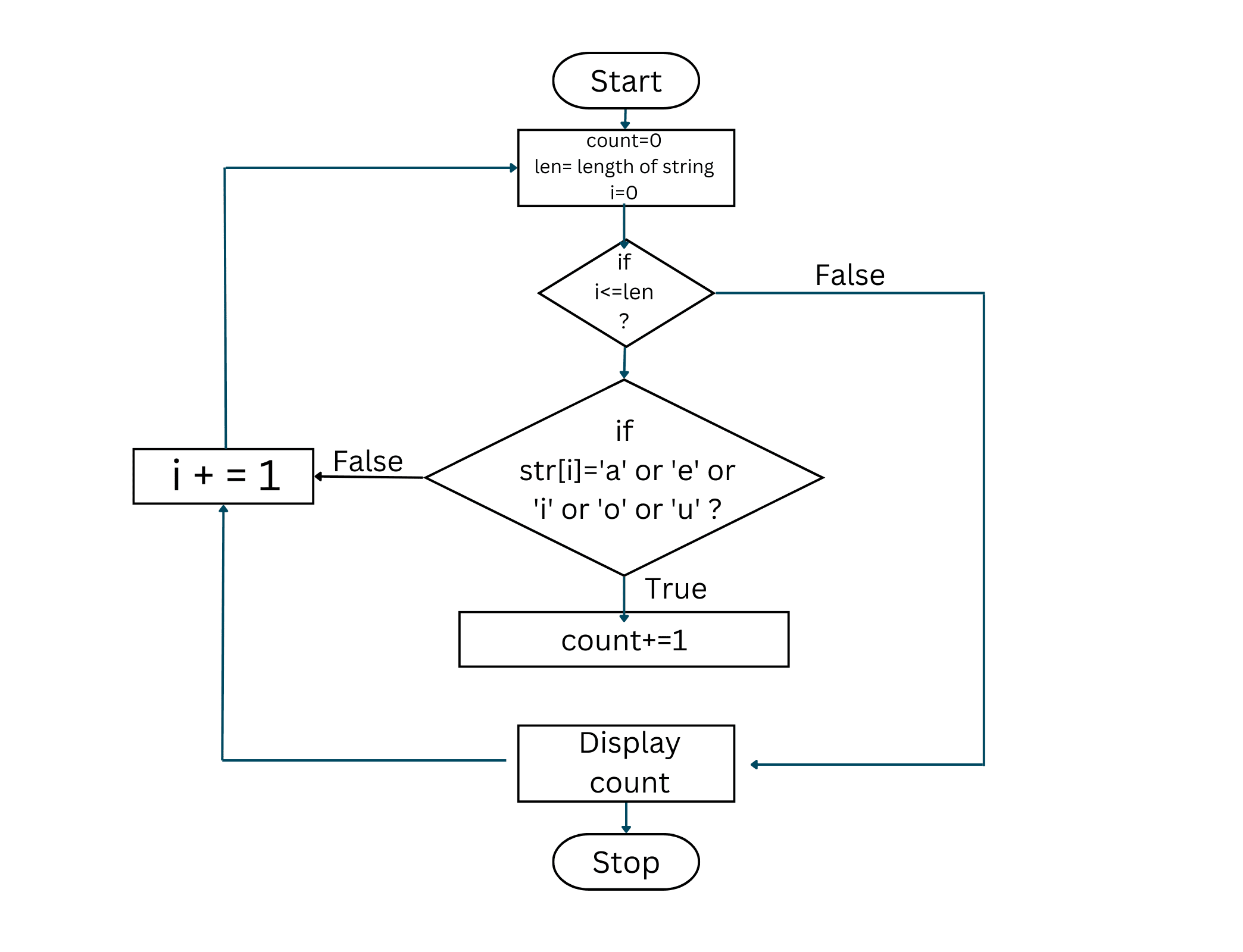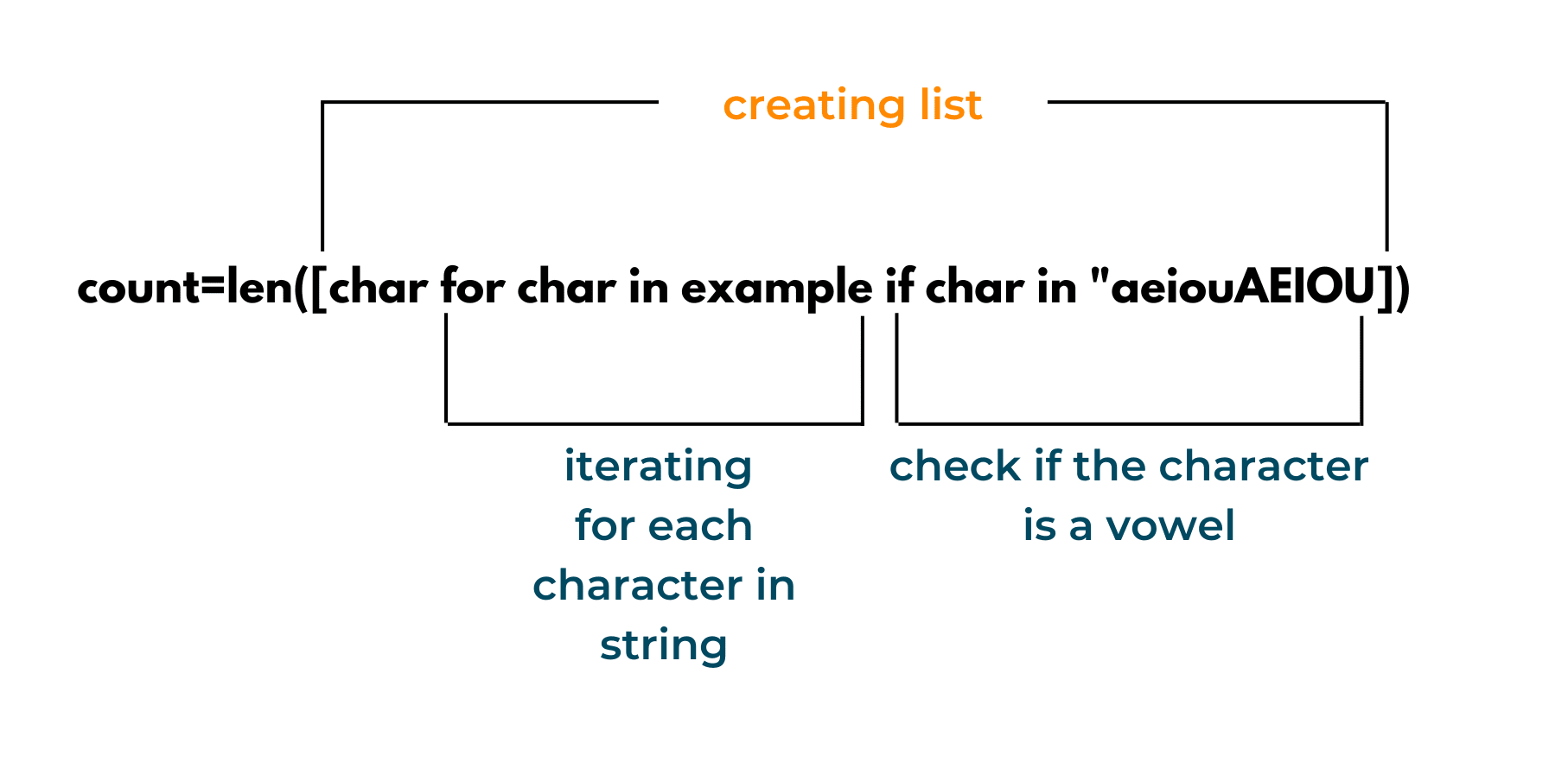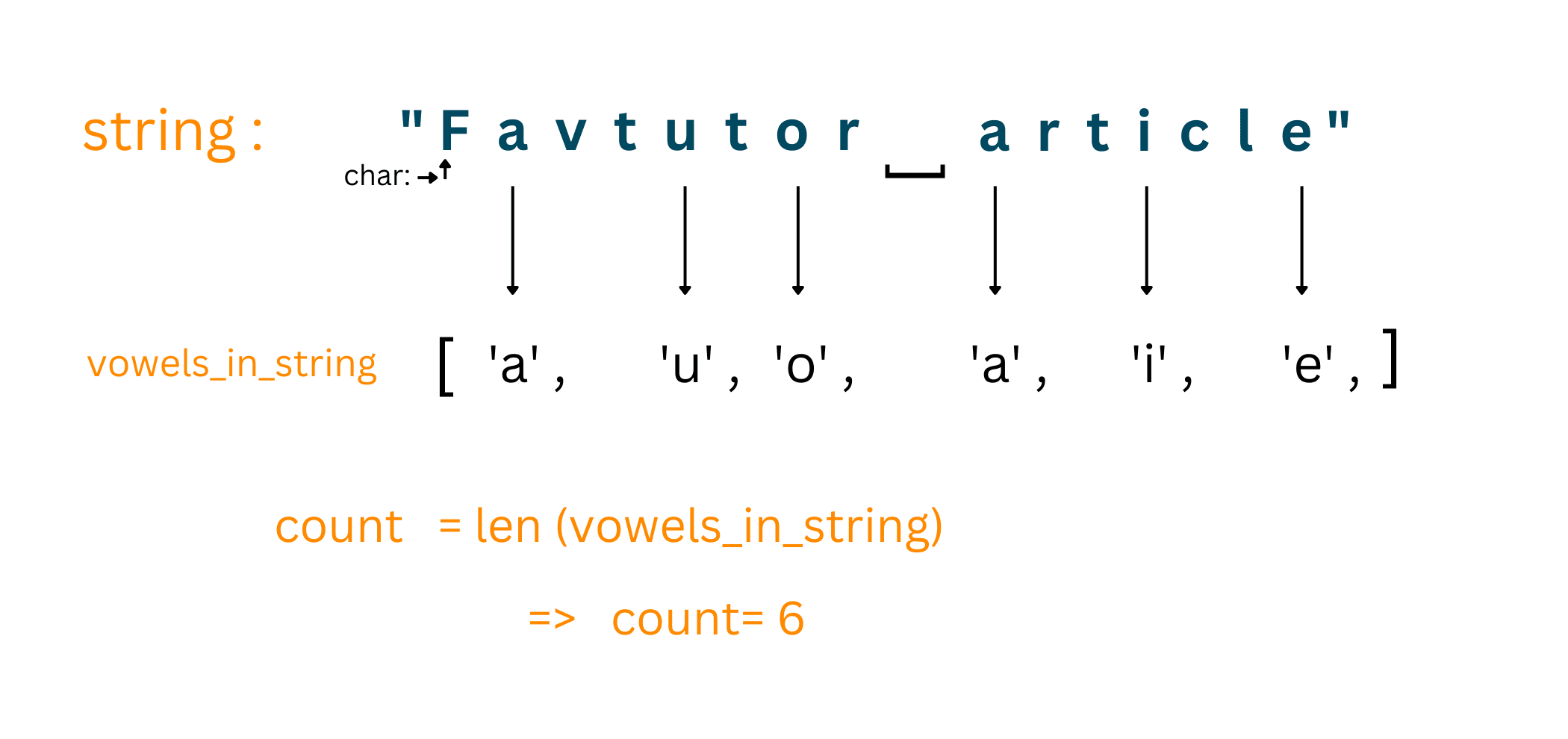# How to Count Vowels in a String using Python? (Loops & Lists)

Strings are an essential factor in programming. Be it online assessments, technical rounds, or interviews, you'll often find problems related to strings in your placement journey. (I dare not say always, but maybe in every round!)

If you're aiming for a MAANG company or any service-based company, you must be well versed with strings since these are always an integrated part of the hiring challenges. Apart from hiring, these are also good starting stones for competitive programming.

Since we're on the topic of hiring challenges, one of the most common questions you'll come across is how to count the number of vowels in a string using Python. Today, we'll be discussing this problem, its variants, and different approaches to solving this problem. So, let's get started!

## How to Count the Number of Vowels in a String?

Here is the problem statement: "You are given a string, made of alphabets, special characters, or numbers. You need to count the number of vowels in the given string."

Sounds like an easy task, right?

There are 5 Vowels: ['a', 'e', 'i', 'o', 'u'].

Since programming languages are generally case-sensitive, hence to determine the number of vowels in a string, you need to consider both the upper case and lower case characters. Hence, you'll have 10 characters in the vowels list as:

['a' , 'e', 'i', 'o', 'u', 'A', 'E', 'I', 'O', 'U']

There are many variations for the given problem statement. Let's begin with the easy one and build our way up to its complexity.

Let's look at an Example:

We are given a string: "Count number of vowels in a String in Python".

Vowels include [ 'a', 'e', 'i', 'o', 'u' ] (for the time being, let's consider only lowercase alphabets)

Refer to the image below for a quick understanding of the problem:Output: 12

Now that you have a basic understanding of the problem, let's move on to our first approach!

### 01) Brute Force Approach

This will be the most basic and your first approach to a problem. Remember that when in an interview, it is helpful, to begin with, a brute force approach. So even if you don't reach the optimal answer, you'll be able to display your logic-building ability and your clear view of the problem.

Also, this helps you clear your ideas at the interview and helps you to explain your solution better to the interviewer.

So, let's move toward our Brute Force Approach for counting the number of vowels in a string,

Algorithm:

1. Take a string as user input
2. Initialize a count variable with 0
3. Iterate over each character of the string
4. Check "IF" the character is a vowel.
5. If the character is a vowel, increment the value of the count variable by 1.
6. Else continue to iterate over the string.
7. Break the loop when the string reaches its end.

Below is a flow chart depicting the flow of the above approach:Since the loop only runs for the length of the string, hence the loop runs specifically n times. Thus, the Time Complexity for the brute force approach is O(n).

Now that you've understood the brute force solution, it's time to put it into action. Let's begin with coding our brute force solution to count vowels in a string using Python:

```# Program: Count number of vowels in a String in Python

example = "Count number of vowels in a String in Python"

# initializing count variable
count = 0

# declaring a variable for index
i = 0

# iterate over the given string (example)
# len(example) -> gives the length of the string in Python

for i in range(len(example)):
if (
(example[i] == "a")
or (example[i] == "e")
or (example[i] == "i")
or (example[i] == "o")
or (example[i] == "u")
):
count += 1

print("Number of vowels in the given string is: ", count)
```

The range() function is used here to increase the value of i by 1 and to check that the value of i lies between 0 and the length of the string.

Output:

```Number of vowels in the given string is:  12
```

The above method was the most basic approach to this problem. Now, let's work together on optimizing this code.

Note that we've used the 'or' operator to compare the character with vowels. You can avoid these many 'or' operators by using the 'in' operator here. Let's check it out!

Optimizing using 'in' operator:

```# Program: Count number of vowels in a String in Python

example = "Count number of vowels in a String in Python"

# initializing count variable
count = 0

# creating a list of vowels
vowels = ["a", "e", "i", "o", "u"]

# iterate over the given string (example)
# len(example) -> gives the length of the string in Python
# Note that python can also declare the variable at the time of calling
for i in range(len(example)):
if example[i] in vowels:
count += 1

print("Number of vowels in the given string is: ", count)
```

Output:

```Number of vowels in the given string is:  12
```

In Python, the 'in' operator determines whether a given value is present in the given sequence (like a string, array, list, or tuple). In the above code, we've created a list storing the vowels. In this way, you can also add the uppercase characters of Vowels to the list.

Hence, the statement ->

`    if example[i] in vowels:`

checks whether the character (example[i]) is present in the list (vowels). This results in 'True' if it is, which is a case for a vowel, else it outputs 'False'.

Instead of lists, you can use any of the sequences here, like - "aeiouAEIOU" or ("aeiou").

Note that in both the approaches above, we've been using the index of the string to iterate over each character. Let's try calling a for-each loop for the purpose.

### 02) Count Vowels in String Using for Loop (as for-each)

A for-each loop is a control flow statement that iterates over elements in a sequence or collection. Unlike other loops, it iterates over all elements rather than keeping a counter, loop variable, or checking a condition after each loop iteration.

In Python, you can use the for loop as a for-each loop. Let's see how:

```# Program: Count number of vowels in a String in Python

# example string
example = "Favtutor article"

vowels = ["a", "e", "i", "o", "u"]
count = 0

# using for-each loop, character is reference to a letter in the string
for character in example:
if character in vowels:
count += 1

print("Number of vowels in the given string is: ", count)
```

Output:

```Number of vowels in the given string is:  6
```

In the above code, the variable 'character' refers to each character in the string 'example'. The loop runs for each character in the string, hence it doesn't actually require a counter variable to iterate over the string.

### 03) Count Vowels in a String with List Comprehension

Can you count the number of vowels in a String using one line of code?

Surprisingly, you can!

Python offers List Comprehensions, which provides a shorter syntax for creating a new list based on the values of an existing list. A Python List Comprehension is made up of brackets containing the expression, which is executed for each element, as well as the for loop, which is used to iterate over the list's elements.

Let's check it out:

```# Program: Count number of vowels in a String in Python

example = "Favtutor article"

# list comprehension
count = len([char for char in example if char in "aeiouAEIOU"])

print("Number of vowels: ", count   )
```

Output:

```Number of vowels:  6
```

Note that we've shortened our whole for loop into one line.

Hard to comprehend? Don't worry, let's go by the list comprehension on breaks.The len() function is used to return the length of the newly created list. It is the len() function which actually gives the count of the number of vowels in the string.

The loop iterates for each character in the string and checks whether 'if' the character is a vowel. If it is, then it appends that character to the list. Hence, in the end, the len() function returns the length of the list which comprises all the vowels in the given string.

Hard to understand?

Let's take a peek behind the scenes of this list comprehension:

The string = "Favtutor article"

Our list comprehension = len([char for char in example if char in "aeiouAEIOU"])

The vowels in the string get appended in the new list. This occurs as we iterate over the string by each character.

Look at the image below for a better understanding-### 04) Using ReGex expression

Python offers the 're module' to easily work with regex expressions. The re module in Python offers a findall() function, that finds the match for a given pattern in the given string.

We can use the findall() function to obtain the count of vowels in the string. Let's take a look at the code:

```# Program: Count number of each vowel in a string in Python

import re

string = "Article: Count number of each vowel in a string in Python"

# using findall() function to match pattern
# Meta character '|' is used to depict 'either-or' sequence
vowels = re.findall("a|e|i|o|u", string)
print(vowels)
# count of number of vowels in the string
print(len(vowels))
```

Output:

```['i', 'e', 'o', 'u', 'u', 'e', 'o', 'e', 'a', 'o', 'e', 'i', 'a', 'i', 'i', 'o']
16
```

### 05) Using 'count()' method

Python provides a count() method to count the number of specified elements in any iterable (like string, list).

Its basic syntax is: variable = iterable_name.count('value')

This returns the count of the specified element in the iterable. You can use the count method with a list comprehension to count the occurrence of each vowel in the string.

Take a look at the below code:

```# Program: Count number of each vowel in a string in Python

string = "Article: Count number of each vowel in a string in Python"

vowels = [string.count(x) for x in "aeiou"]
# the produced list
print(vowels)

# count of vowels in the string
print(sum(vowels))
```

Output:

```[2, 4, 4, 4, 2]
16
```

Note that the vowels (list) hold the number of each vowel in the string in the given sequence (aeiou).

## Conclusion

In the above article, you've learned how to count the number of vowels in a string in Python. Note that since I've only used the small-case vowels ('aeiou') in the above examples, the above code will not consider upper-case alphabets ('AEIOU') while counting the vowels.

You'll need to mention the upper-case vowels ('AEIOU') separately for them to be counted as well. You can use the lowercase() or uppercase() function over the given string to convert the letters to the same case, to obtain more efficient results.

Apart from the above-mentioned methods, you'll find other methods that'll do the task for you. You'll have to optimize the code and use the method with the minimum time complexity for better results.

Happy Coding!

### FavTutor - 24x7 Live Coding Help from Expert Tutors!